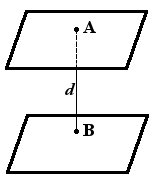Study of mathematics online.
Study math with us and make sure that "Mathematics is easy!"

# Distance between two planesDefinition. The distance between two planes is equal to length of the perpendicular lowered from a point on a plane.

## Distance between two planes formula

If Ax + By + Cz + D1 = 0 and Ax + By + Cz + D2 = 0 is a plane equation, then distance between planes can be found using the following formula

 d = |D2 - D1| √A2 + B2 + C2

## Examples of tasks with distance between two planes

Example 1.
To find distance between planes 2x + 4y - 4z - 6 = 0 and x + 2y - 2z + 9 = 0.

Solution. Let's check up, whether planes are parallel, for this purpose we will multiply the equation of the second plane on 2

2x + 4y - 4z + 18 = 0

As planes are parallel than for calculation distance between planes we use the formula:

 d = |18 - (-6)| = |24| = 24 = 4 √22 + 42 + (-4)2 √36 6

Answer: distance from plane to plane is equal to 4.